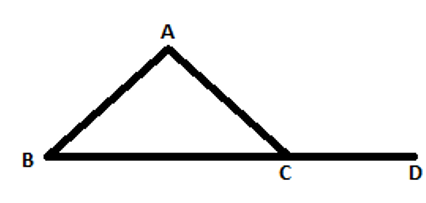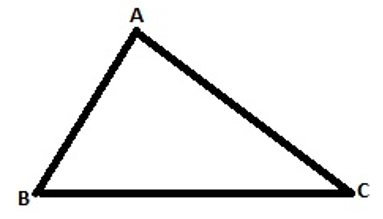# Triangle Inequalities Worksheets

What Are Triangle Inequalities? Triangle is one of the smallest possible polygons with three sides and three angles the classification of triangles is based on the measurements of its angles and length of sides. The inequality theorem can be applied to every type of triangle. An exterior angle is the one that is formed between any side and the extension of the adjacent side of the triangle. We can from six exterior angles, two at each vertex. The exterior angle inequality theorem states that the measure of any exterior angle of a triangle is greater than both of the non-adjacent interior angles. All six types of triangle satisfy this theorem.In the above figure, we can see that angle ACD is drawn as the exterior angle. And m∠ACD > m∠CAB and m∠ACD > m∠CBA . Triangle Inequalities Theorem - The triangle cannot be formed by any set of random measurements. The triangle inequality theorem states that the sum of lengths two sides of the triangle will always be greater than the length of the third side. It means that if we are given two sides of a triangle, we can safely say that the length of the third side will be smaller than the sum of those given lengths.In the figure shown above, we have three inequalities, AB + BC > AC | BC + AC > AB | And AB + AC > BC.

• ### Basic Lesson

Guides students through solving Triangle Inequalities. In triangle PQR, an exterior angle at P measures 140°, and m∠ Q = 60°. Which is the longest side of the triangle?

• ### Intermediate Lesson

Demonstrates the concept of advanced skill while solving Triangle Inequalities. The lengths of two sides of a triangle ABC are 6, 10. Find the possible lengths of the third side.

• ### Independent Practice 1

A really great activity for allowing students to understand the concepts of the Triangle Inequalities.

• ### Independent Practice 2

Students use Triangle Inequalities in 20 assorted problems. The answers can be found below.

• ### Homework Worksheet

Students are provided with 12 problems to achieve the concepts of Triangle Inequalities.

• ### Skill Quiz

This tests the students ability to understand Triangle Inequalities.